# How to Use the Math Editor

This is a guide for using the Math Editor. The Math Editor allows you to build simple to complex equations using LaTex that look great.

Accessing the Math Editor

2. Create a new modality or customize existing content.
3. In the editor, locate the math editor icon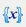4. Click on the icon to open the math editor.

Using the Equation templates1. Click on any of the common equations in the menu to select an equation.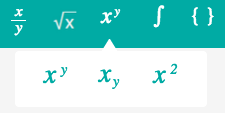1. Type in numbers in the input boxes to start building an equation.

EX: XY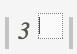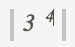1. Continue building your equation using the options from the menu or typing in numbers or letters as necessary.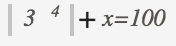1. Click Preview to render the equation in the preview box.
1. When finished, click the Add button in the bottom right corner to insert the equation.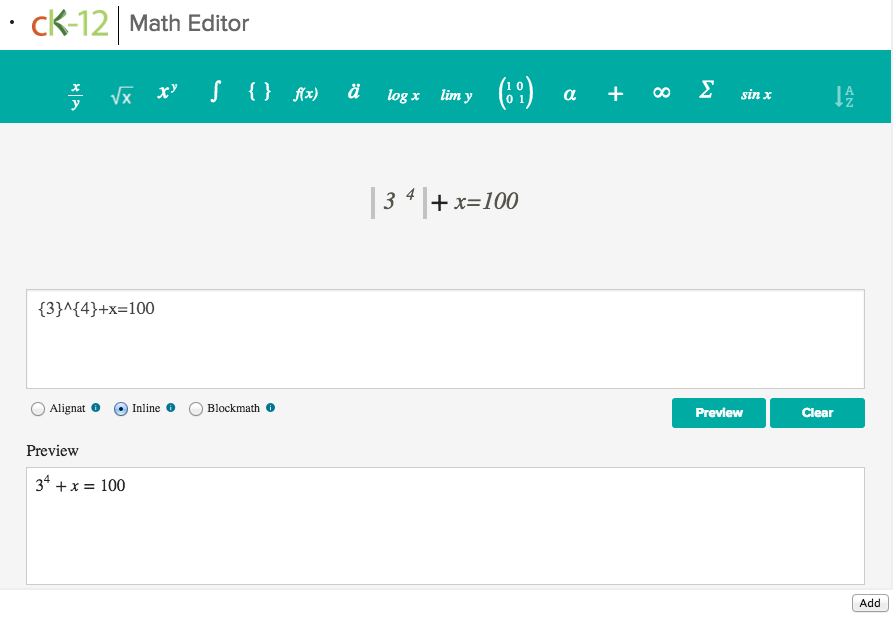Typing LaTex directly

You can input LaTex code directly into the math editor in the 1st input box.

1. Enter your LaTex equation into the 1st input box.

Ex: \frac{3}{x-2}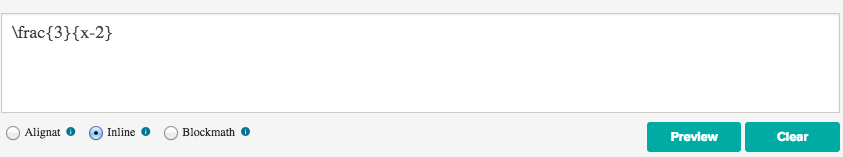1. Click Preview to render your changes in the preview.
2. When finished, click the Add button in the bottom right corner to insert the equation.